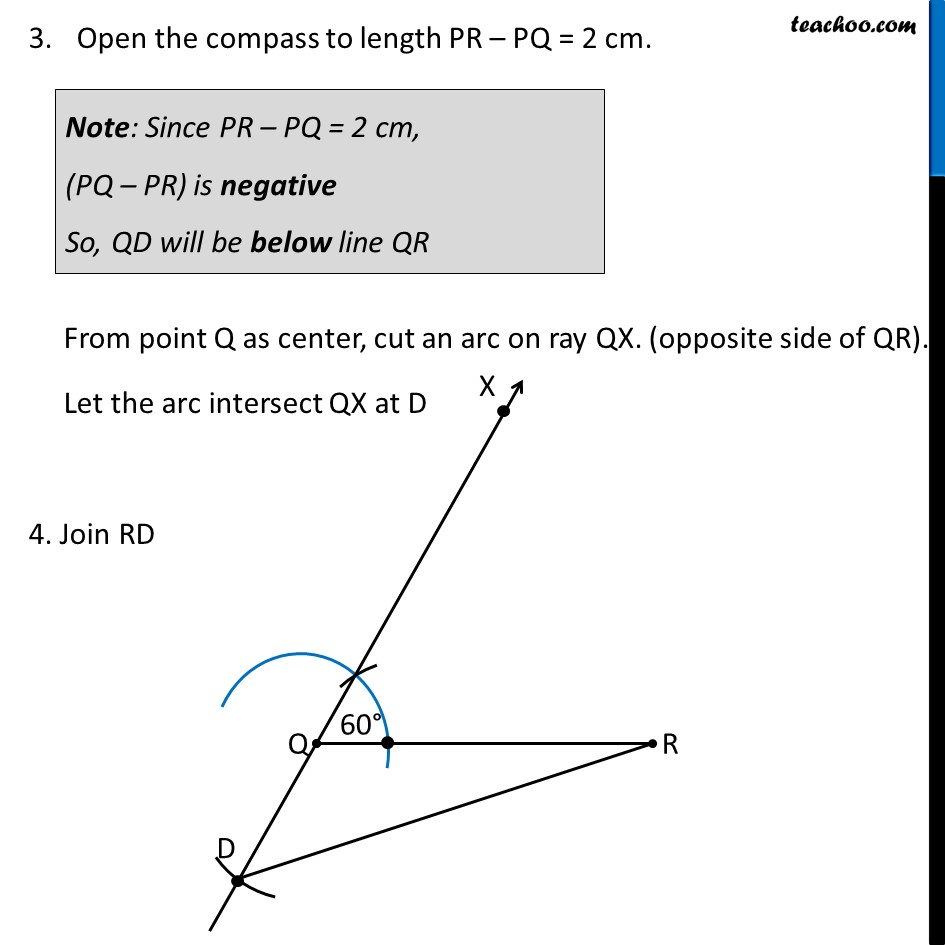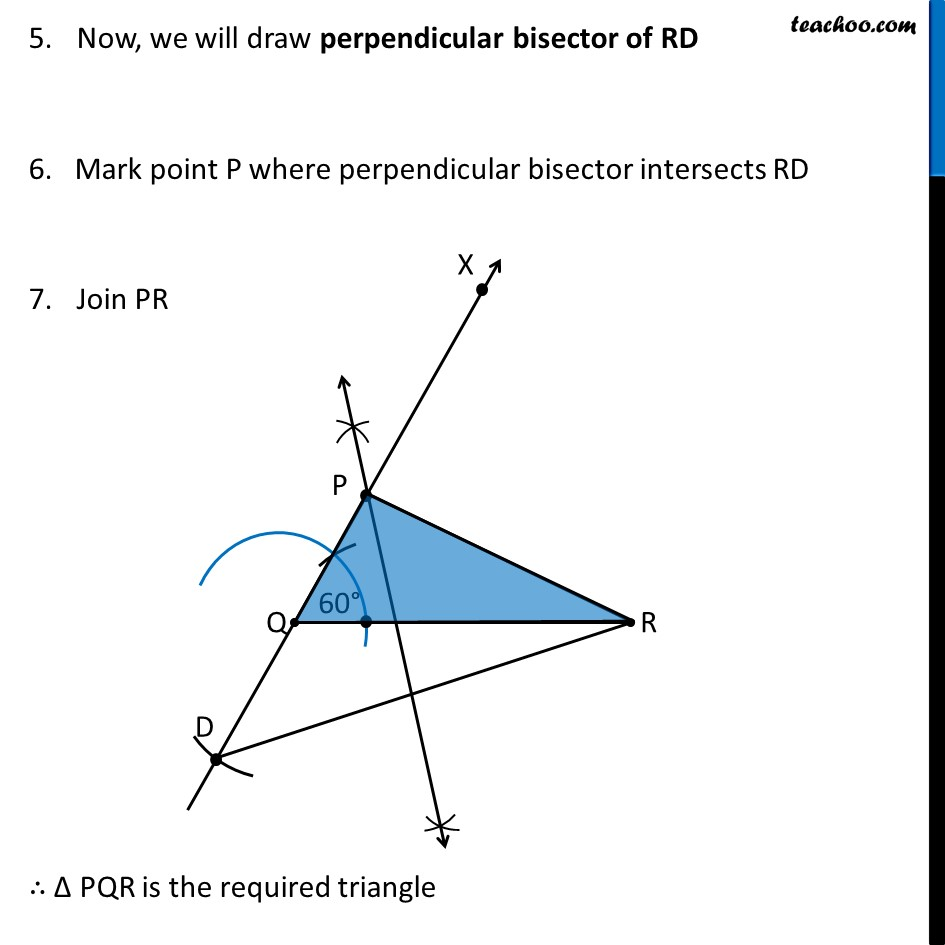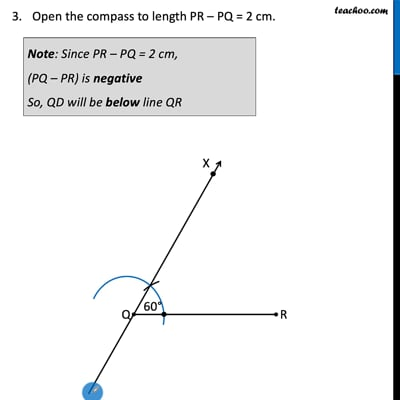Constructing Triangle - Given base, angle and difference of sidesThis video is only available for Teachoo black users

Introducing your new favourite teacher - Teachoo Black, at only ₹83 per month

### Transcript

Ex 11.2, 3 Construct a triangle PQR in which QR = 6 cm, ∠Q = 60° and PR − PQ = 2 cm. Steps of Construction: Draw base QR of length 6 cm 2. Now, let’s draw ∠ Q = 60° Let the ray be QX Open the compass to length PR – PQ = 2 cm. From point Q as center, cut an arc on ray QX. (opposite side of QR). Let the arc intersect QX at D 4. Join RD Note: Since PR – PQ = 2 cm, (PQ – PR) is negative So, QD will be below line QR Now, we will draw perpendicular bisector of RD 6. Mark point P where perpendicular bisector intersects RD Join PR ∴ Δ PQR is the required triangle